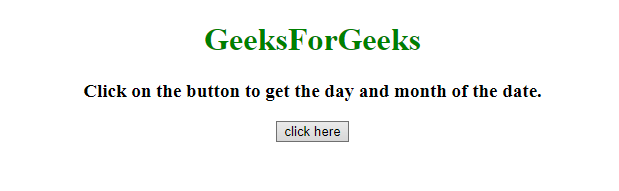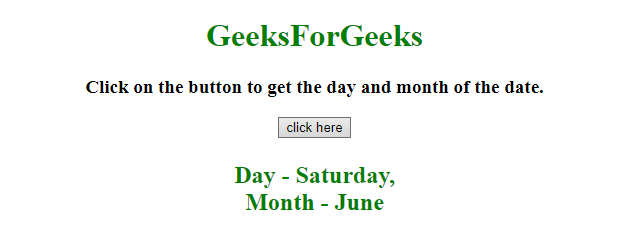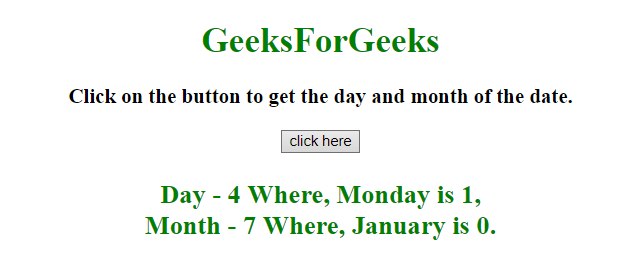Related Articles

# How to get the day and month of a year using JavaScript ?

• Last Updated : 23 Aug, 2019

Given a date and the task is to get the day and month of a year using JavaScript.

Approach:

• First get the current date by using new Date().
• Use currentDate.getDay() to get the current day in number format, Map it to the day name.
• Use currentDate.getMonth() to get the current month in number format, Map it to the month name.

Example 1: In this example, the month and date is determined by the above approach.

 ` ``<``html``> `` ` `<``head``> ``    ``<``title``> ``        ``How to get the day and month``        ``of a year using JavaScript ?``    ```` `` ` `<``body` `style` `= ``"text-align:center;"``> ``     ` `    ``<``h1` `style` `= ``"color:green;"` `> ``        ``GeeksForGeeks``    ````     ` `    ``<``p` `id` `= ``"GFG_UP"` `style` `=``        ``"font-size: 19px; font-weight: bold;"``>``    ````     ` `    ``<``button` `onclick` `= ``"GFG_Fun()"``>``        ``click here``    ````     ` `    ``<``p` `id` `= ``"GFG_DOWN"` `style` `=``        ``"color: green; font-size: 24px; font-weight: bold;"``>``    ````     ` `    ``<``script``>``        ``var el_up = document.getElementById("GFG_UP");``        ``var el_down = document.getElementById("GFG_DOWN");``         ` `        ``el_up.innerHTML = "Click on the button to get "``                ``+ "the day and month of the date.";``         ` `        ``var Days = ['Sunday', 'Monday', 'Tuesday', ``                ``'Wednesday', 'Thursday', 'Friday', 'Saturday'];`` ` `        ``var Months = ['January', 'February', 'March', 'April', ``                ``'May', 'June', 'July', 'August', 'September',``                ``'October', 'November', 'December'];``         ` `        ``var currentDay = new Date();``         ` `        ``// Get the current day name``        ``var day = Days[currentDay.getDay()];``         ` `        ``// Get the current month name``        ``var month = Months[currentDay.getMonth()]; ``         ` `        ``function GFG_Fun() {``            ``el_down.innerHTML = "Day - " + day``                    ``+ ",<``br``> Month - " + month;``        ``}``    `` `` `` ` ``

Output:

• Before clicking on the button:• After clicking on the button:Example 2: This is same example but with a different approach. In this example, the month and date is determined by the above approach.

 ` ``<``html``> `` ` `<``head``> ``    ``<``title``> ``        ``How to get the day and month of``        ``a year using JavaScript ?``    ```` `` ` `<``body` `style` `= ``"text-align:center;"``> ``     ` `    ``<``h1` `style` `= ``"color:green;"` `> ``        ``GeeksForGeeks``    ````     ` `    ``<``p` `id` `= ``"GFG_UP"` `style` `=``        ``"font-size: 19px; font-weight: bold;"``>``    ````     ` `    ``<``button` `onclick` `= ``"GFG_Fun()"``>``        ``click here``    ````     ` `    ``<``p` `id` `= ``"GFG_DOWN"` `style` `=``        ``"color: green; font-size: 24px; font-weight: bold;"``>``    ````     ` `    ``<``script``>``        ``var el_up = document.getElementById("GFG_UP");``        ``var el_down = document.getElementById("GFG_DOWN");``     ` `        ``el_up.innerHTML = "Click on the button to get the "``                    ``+ "day and month of the date.";``         ` `        ``var currentDay = new Date();``         ` `        ``// Get the current day name``        ``var day = currentDay.getDay(); ``         ` `        ``// Getting the current month name``        ``var month = currentDay.getMonth(); ``         ` `        ``function GFG_Fun() {``            ``el_down.innerHTML = "Day - " + day + ``                    ``" Where, Monday is 1,<``br``> Month - "``                    ``+ month +" Where, January is 0.";``        ``}``    `` `` `` ` ``

Output:

• Before clicking on the button:• After clicking on the button:Hey geek! The constant emerging technologies in the world of web development always keeps the excitement for this subject through the roof. But before you tackle the big projects, we suggest you start by learning the basics. Kickstart your web development journey by learning JS concepts with our JavaScript Course. Now at it’s lowest price ever!

My Personal Notes arrow_drop_up